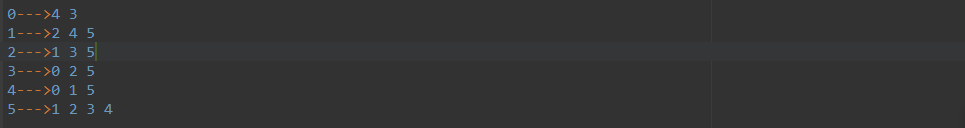### C++: Program to implement Graph using Adjacency List

Graph Data Structure
Introduction :

The adjacency list representation of a graph is linked list representation. In this representation we have an array of lists The array size is V. Here V is the number of vertices. In other words, we can say that we have an array to store V number of different lists. If a list header is vertex u, then it signifies that it will hold all of the adjacent vertices of u.

### This representation takes O(V+2E) for undirected graph, and O(V+E) for directed graph. If the number of edges are increased, then the required space will also be increased.

Input: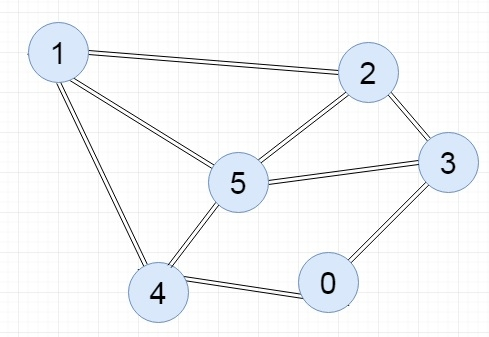Output: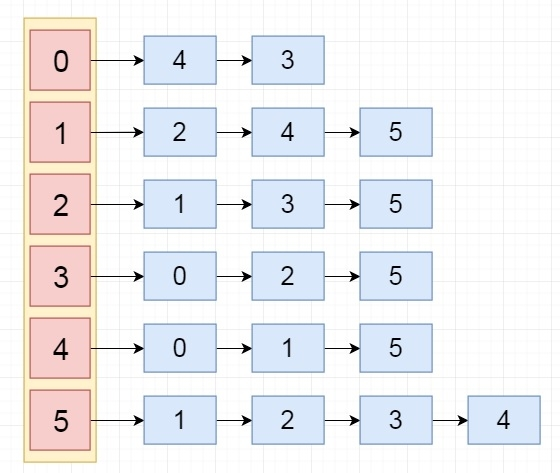Program to implement Graph in Adjacency List in C++: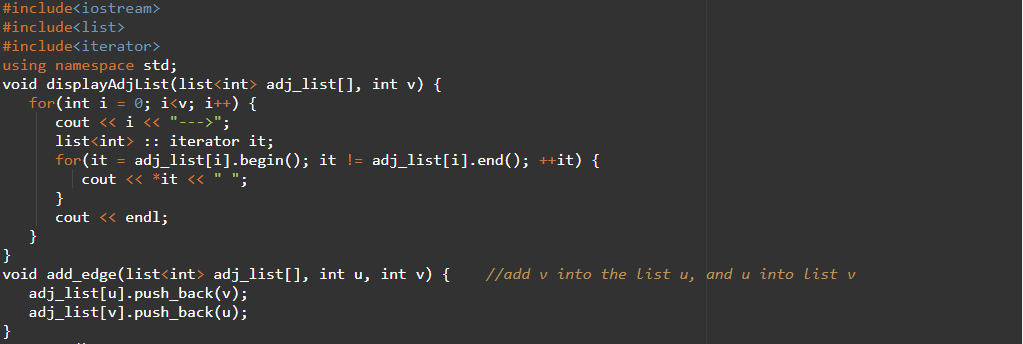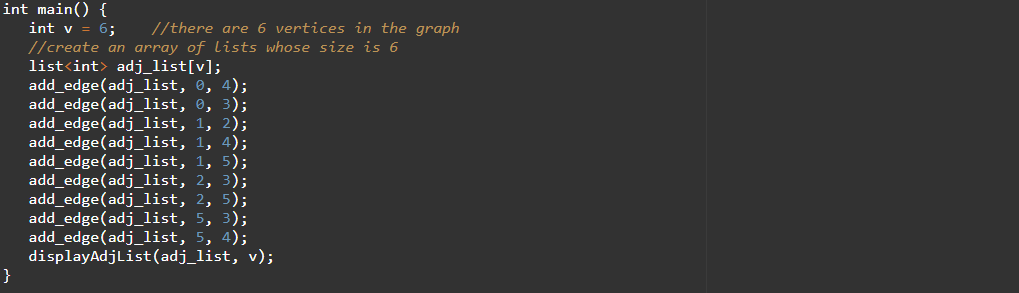OUTPUT: